# Bayesian Posterior Sampling¶

When using Bayesian posterior sampling, we want to the posterior, $${\rm P}(M(\vec{\theta}) \vert D)$$, of the model ($$M(\vec{\theta})$$) given one or more datasets ($$D$$) (i.e., plugin instances) and one model containing one or more sources with free parameters $:nbsphinx-math:vec{theta}$ given a likelihood $$L(\vec{\theta}) = {\rm P}(D \vert M(\vec{\theta}))$$ and prior $${\rm P}(\theta)$$ on the parameters. Only the simplest posteriors allow for an analytic solution, thus we must rely on Monte Carlo or nested sampling methods to sample the posterior.

In 3ML, we currently provide two popular posterior sampling methods: emcee and MULTINEST. If you installed via conda, both packages are available, otherwise, only emcee is included.

## emcee¶

emcee is an extensible, pure-Python implementation of Goodman & Weare’s Affine Invariant Markov chain Monte Carlo (MCMC) Ensemble sampler.” It uses multiple “walkers” to explore the parameter space of the posterior. For a complete understanding of the capabilites and limitations, we recommend a thorough reading of Goodman & Weare (2010). Nevertheless we emphasize these N points to keep in mind:

• emcee is for unimodal parameter estimation

• for complex likelihoods and marginal likelihood integration, check out thermodynamic integration (documentation coming soon)

Let’s take a look at its usage for a simple likelihood.

:

%matplotlib notebook
import matplotlib.pyplot as plt
from threeML import *
from threeML.bayesian.tutorial_material import *


Let’s get a BayesianAnalysis object like the one we would have in a normal 3ML analysis. We use a custom function, prepared for this tutorial, which gives a BayesianAnalysis object having a very simple model with one free parameter ($$\mu$$), and with a likelihood having a very simple shape:

:

# This returns a BayesianAnalysis object with a simple likelihood function,
# and the corresponding Model instance. These objects are what you will have
# in a typical 3ML analysis. The Model contains one point source, named "test",
# with a spectrum called "simple"
bayes, model = get_bayesian_analysis_object_simple_likelihood()
bayes.set_sampler('emcee')
# Let's look at the likelihood function, which in this illustrative example
# has a very simple shape
_ = plot_likelihood_function(bayes)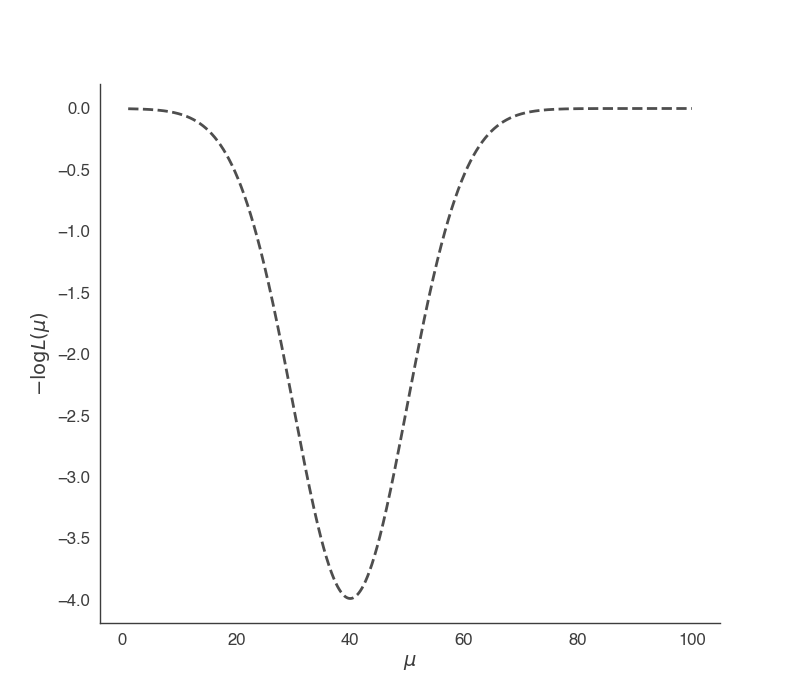We must define a prior fo $$\mu$$ in order to sample. Let’s use a uniform prior from 0 to 100. There are two ways to define this in 3ML:

:

# This directly assigns the prior
model.test.spectrum.main.shape.mu.prior = Uniform_prior(lower_bound=1, upper_bound=100.)

# Alternatively, we can set an uniformative prior on the parameter's bounds
model.test.spectrum.main.shape.mu.set_uninformative_prior(Uniform_prior)



Ok, we are ready to sample. We will use only 5 walkers for this problem. We need to define a burn_in sample length which is how long we want to run the sampler to approach the target distribution so that we do not include these “learning” samples in our final results. Additionally, we need to declare the number of samples each walker will take.

:

n_walkers = 5
burn_in = 10
n_samples = 500

bayes.sampler.setup(n_iterations=n_samples,n_burn_in=burn_in,n_walkers=n_walkers )

res = bayes.sample()

100%|██████████| 10/10 [00:00<00:00, 949.04it/s]
100%|██████████| 500/500 [00:00<00:00, 1408.44it/s]


Mean acceptance fraction: 0.742

Maximum a posteriori probability (MAP) point:


result unit
parameter
test.spectrum.main.Simple.mu (3.8 -1.0 +0.8) x 10 keV

Values of -log(posterior) at the minimum:


-log(posterior)
custom 3.989422
total 3.989422

Values of statistical measures:


statistical measures
AIC -9.978844
BIC -7.978844
DIC -3.750087
PDIC 2.016456
:

_ = plot_sample_path(bayes,truth=40.,burn_in=n_walkers*burn_in)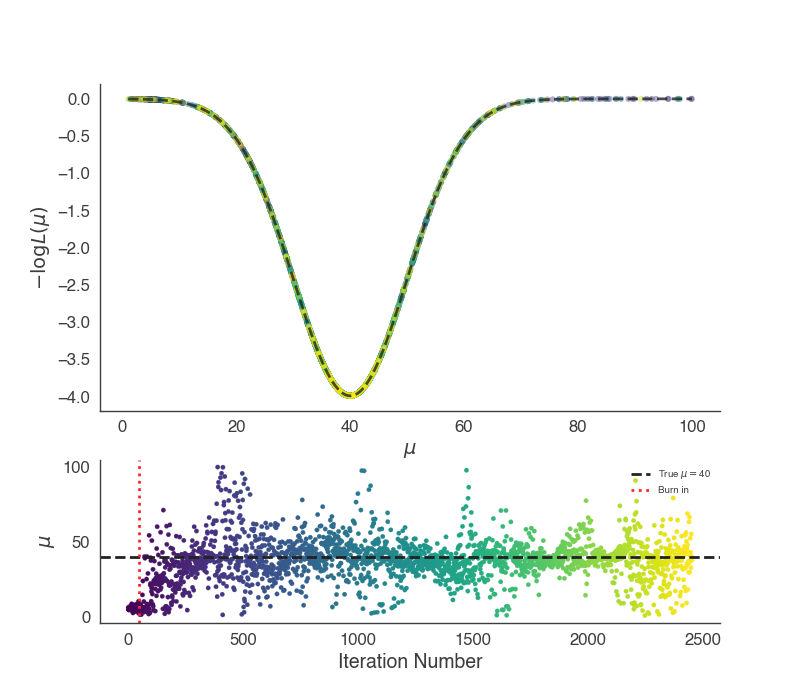We can see that we did not burn in the sampler long enough and we will have part of the burn in included in the results as can be seen in the marginal distribution of $$\mu$$:

:

_ = bayes.results.corner_plot()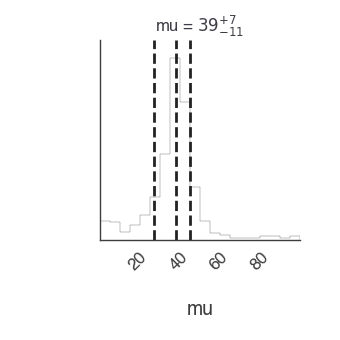We could easily run the sampler longer, but let’s reset the value of $$\mu$$ far from the target distribution and try more burn in samples.

:

model.test.spectrum.main.shape.mu =99

n_walkers = 2
burn_in = 200
n_samples = 500

res = bayes.sample()

100%|██████████| 10/10 [00:00<00:00, 1002.58it/s]
100%|██████████| 500/500 [00:00<00:00, 1380.83it/s]


Mean acceptance fraction: 0.7407999999999999

Maximum a posteriori probability (MAP) point:


result unit
parameter
test.spectrum.main.Simple.mu (4.0 -0.9 +0.7) x 10 keV

Values of -log(posterior) at the minimum:


-log(posterior)
custom 3.989423
total 3.989423

Values of statistical measures:


statistical measures
AIC -9.978845
BIC -7.978845
DIC -3.738946
PDIC 2.118753
:

_ = plot_sample_path(bayes,truth=40.,burn_in=n_walkers*burn_in)
_ = bayes.results.corner_plot()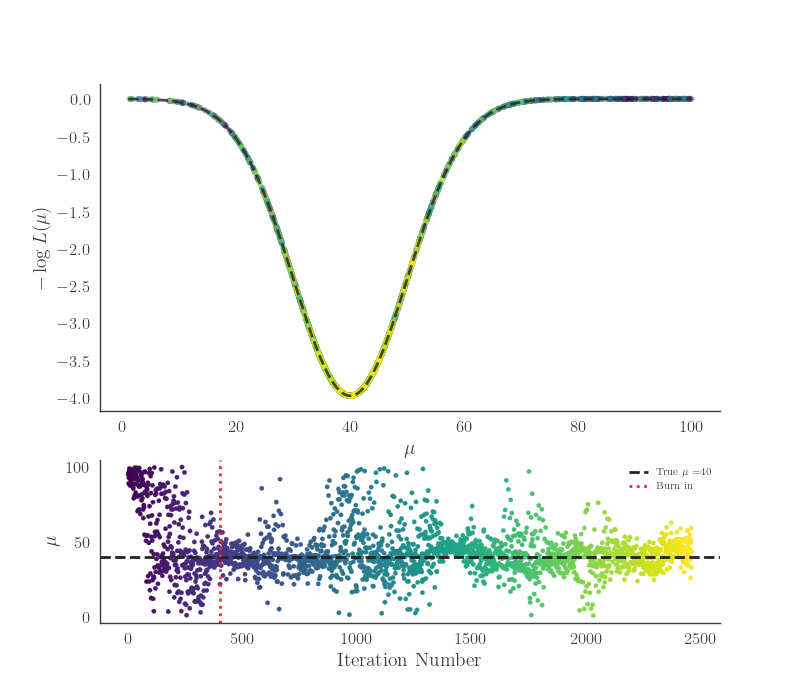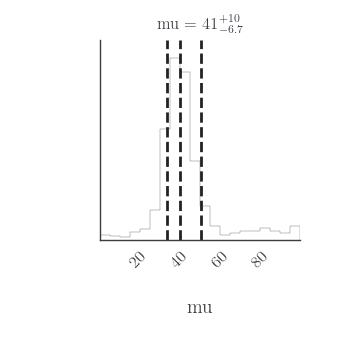The marginal distribution of the parameter $$\mu$$ is the answer for our parameter. It is the integral of the posterior over all other parameters, $$\vec{\phi}$$, given the data.

${\rm P}(\mu \vert D) = \int_{-\infty}^{\infty} {\rm d}\vec{\phi} \; {\rm P}( \mu, \vec{\phi}\vert D )$

Of course, in our simple model, there are no other parameters. The marginal natuarally includes the dependence of all other parameters and is only equivalent to MLE error estimates under assumptions of Gaussianity and linearity.

We can sometimes use point-descriptors for the marginal, e.g., mean, median, etc., but when the marginal distribution is not easily symmetric, it can be more descriptive to plot it or speak of its highest posterior density interval:

:

this_mu = bayes.results.get_variates('test.spectrum.main.Simple.mu')

print(this_mu.highest_posterior_density_interval(cl=.68))
print(this_mu.highest_posterior_density_interval(cl=.95))

(31.67224005194367, 46.98179350848535)
(1.0258270433669878, 65.17269053666413)


### Priors¶

We have not paid much attention to our prior choice. So far, we have used an uninformative prior (except that we know in our example the range over which the parameter can be found), but what happens if we limit our prior such that we would never find the target distribution?

Let’s set a uniform prior such that $$\mu \in \{80-100 \}$$.

:

model.test.spectrum.main.shape.mu =99
model.test.spectrum.main.shape.mu.prior = Uniform_prior(lower_bound=80, upper_bound=100)

n_walkers = 2
burn_in = 200
n_samples = 1000

bayes.sampler.setup(n_iterations=n_samples,n_burn_in=burn_in,n_walkers=n_walkers )
res = bayes.sample()

100%|██████████| 200/200 [00:00<00:00, 2024.94it/s]
100%|██████████| 1000/1000 [00:00<00:00, 2141.53it/s]


Mean acceptance fraction: 0.842

Maximum a posteriori probability (MAP) point:




result unit
parameter
test.spectrum.main.Simple.mu (9.0 +/- 0.7) x 10 keV

Values of -log(posterior) at the minimum:


-log(posterior)
custom 0.001338
total 0.001338

Values of statistical measures:


statistical measures
AIC -2.002676
BIC -0.002676
DIC -0.000638
PDIC -0.000302
:

_ = plot_sample_path(bayes,truth=40.,burn_in=n_walkers*burn_in)
_ = bayes.results.corner_plot()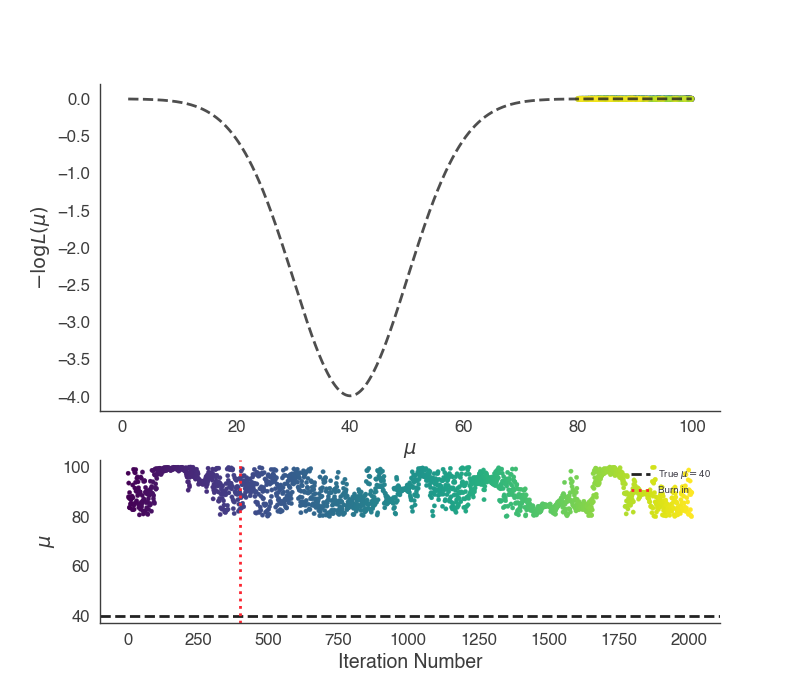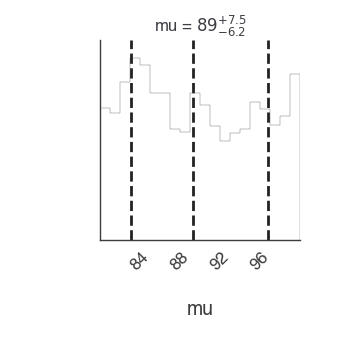We see that the over this range, the marginal distribution is the same as the prior, i.e., uniformative. The “data” have not updated our information about $$\mu$$ and thus we cannot conclude anything. This is by design in our example, but if we were to believe that $$\mu$$ had to be found in the interval $$\mu \in\{80-100\}$$, the data have not helped us to determine anything.

Let’s now look at setting a more informative prior on $$\mu$$. Suppose from either other measurements or physical insight, we are sure $$\mu\sim 30$$ with some uncertainty. Then we can impose a Gaussian prior over $$\mu$$.

:

model.test.spectrum.main.shape.mu =99
model.test.spectrum.main.shape.mu.bounds = (None, None)
model.test.spectrum.main.shape.mu.prior = Gaussian(mu=25,sigma=5)

:

n_walkers = 2
burn_in = 200
n_samples = 1000

bayes.sampler.setup(n_iterations=n_samples,n_burn_in=burn_in,n_walkers=n_walkers )
res = bayes.sample()

100%|██████████| 200/200 [00:00<00:00, 1856.99it/s]
100%|██████████| 1000/1000 [00:00<00:00, 2009.05it/s]


Mean acceptance fraction: 0.8009999999999999

Maximum a posteriori probability (MAP) point:


result unit
parameter
test.spectrum.main.Simple.mu (3.5 -0.6 +0.5) x 10 keV

Values of -log(posterior) at the minimum:


-log(posterior)
custom 1.554065
total 1.554065

Values of statistical measures:


statistical measures
AIC -5.108131
BIC -3.108131
DIC -0.606144
PDIC 1.250983

Our prior information has biased the results to slightly lower values of $$\mu$$. Again, this is only to illustrate how to use different priors and their effects on the recovered results.

:

_ = plot_sample_path(bayes,truth=40.,burn_in=n_walkers*burn_in)
_ = bayes.results.corner_plot()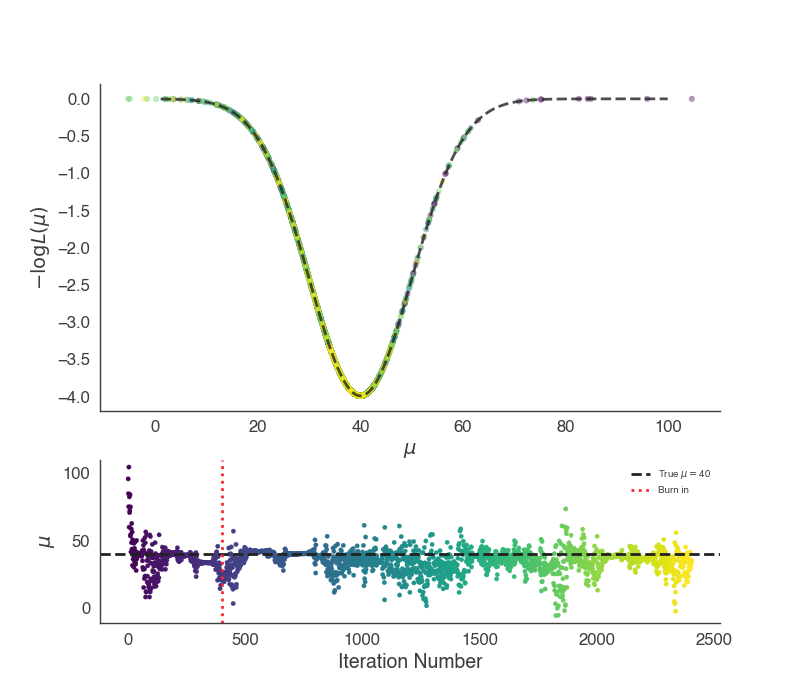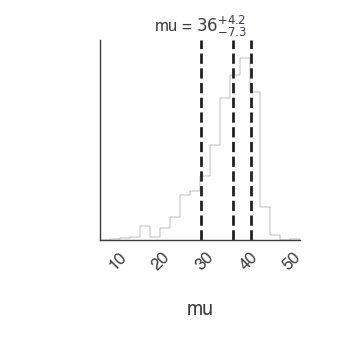## The Zeus sampler¶

We now examine a more complex likelihood with zeus. Zeus builds upon the approach of emcee and by adding on slice sampling.

:

bayes, model = get_bayesian_analysis_object_complex_likelihood()

bayes.set_sampler('zeus')

model.test.spectrum.main.shape.mu.prior = Uniform_prior(lower_bound=1, upper_bound=100)

_ = plot_likelihood_function(bayes)